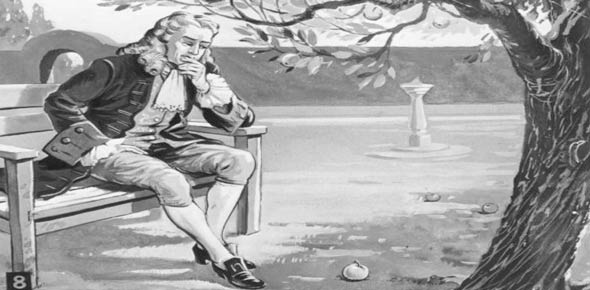# Newton's Laws Of Motion

10 Questions | Total Attempts: 74Settings• 1.
Swimming is possible on account of
• A.

First law of motion

• B.

Second law of motion

• C.

Third law of motion

• D.

Newton's law of gravitation

• 2.
A man of weight 75 kg is standing in an elevator which is moving with an acceleration of 5 m/s in upward direction the apparent weight of the man will be (g=10)
• A.

1425 N

• B.

1250 N

• C.

1375 N

• D.

1125 N

• 3.
Rocket engines lift a rocket from the earth surface because hot gas with high velocity
• A.

Push against the earth

• B.

React against the rocket and push it up

• C.

Push against the air

• D.

Heat up the air which lifts the rocket

• 4.
A rope of length 5m is kept on frictionless surface and a force of 5N is applied to one of its end. Find tension in the rope at 1m from this end
• A.

1 N

• B.

4 N

• C.

5 N

• D.

3 N

• 5.
A body of mass 2 kg moving on a horizontal surface with an initial velocity of 4 m/sec comes to rest after 2 sec. If one wants to keep this body moving on the same surface with a velocity of 4 m/sec, the force required is
• A.

8 N

• B.

Zero

• C.

4 N

• D.

2 N

• 6.
A parachutist of weight ‘w’ strikes the ground with his legs fixed and comes to rest with an upward acceleration of magnitude 3 g. Force exerted on him by ground during landing is
• A.

W

• B.

3w

• C.

2w

• D.

4w

• 7.
A spring balance is attached to the ceiling of a lift. A man hangs his bag on the spring and the spring reads 49 N, when the lift is stationary. If the lift moves downward with an acceleration of 5m/s2 the reading of the spring balance will be
• A.

49 N

• B.

74 N

• C.

24 N

• D.

15 N

• 8.
If force on a rocket having exhaust velocity of 300 m/sec is 210 N, then rate of combustion of the fuel is
• A.

0.7 kg/s

• B.

0.07 kg/s

• C.

1.4 kg/s

• D.

10.7 kg/s

• 9.
A light spring balance hangs from the hook of the other light spring balance and a block of mass M kg hangs from the former one. Then the true statement about the scale reading is
• A.

Both the scales read M/2 kg each

• B.

The scale of the lower one reads M kg and of the upper one zero

• C.

Both the scales read M kg each

• D.

The reading of the two scales can be anything but the sum of the reading will be M kg

• 10.
Gravels are dropped on a conveyor belt at the rate of 0.5 kg/sec. The extra force required in newtons to keep the belt moving at 2 m/sec is.
• A.

1

• B.

4

• C.

2

• D.

0.5

Related TopicsBack to top